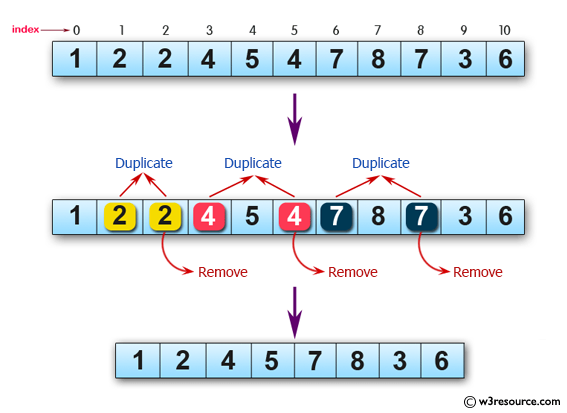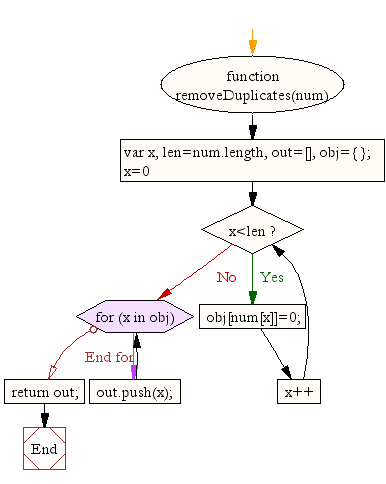# JavaScript: Remove duplicate items from an array, ignore case sensitivity

## JavaScript Array: Exercise-14 with Solution

Write a JavaScript program to remove duplicate items from an array (ignore case sensitivity).

Pictorial Presentation:Sample Solution-1:

JavaScript Code:

``````function removeDuplicates(num) {
var x,
len=num.length,
out=[],
obj={};

for (x=0; x<len; x++) {
obj[num[x]]=0;
}
for (x in obj) {
out.push(x);
}
return out;
}
var Mynum = [1, 2, 2, 4, 5, 4, 7, 8, 7, 3, 6];
result = removeDuplicates(Mynum);
console.log(Mynum);
console.log(result);
```
```

Sample Output:

```[1,2,2,4,5,4,7,8,7,3,6]
["1","2","3","4","5","6","7","8"]
```

Flowchart:Sample Solution-2:

Removing duplicates from an array in JavaScript can be done in a variety of ways, such as using Array.prototype.reduce(), Array.prototype.filter() or even a simple for loop. But there's an easier alternative. JavaScript's built-in Set object is described as a collection of values, where each value may occur only once. A Set object is also iterable, making it easily convertible to an array using the spread (...) operator.

JavaScript Code:

``````//Source:https://bit.ly/3hEZdCl
//Remove duplicates from a JavaScript array
const nums = [1, 2, 2, 3, 1, 2, 4, 5, 4, 2, 6];
console.log([...new Set(nums)])
```
```

Sample Output:

```[1,2,3,4,5,6]
```

Live Demo:

See the Pen JavaScript - Remove duplicate items from an array, ignore case sensitivity - array-ex- 14 by w3resource (@w3resource) on CodePen.

What is the difficulty level of this exercise?

﻿

## JavaScript: Tips of the Day

getList function

```const getList = ([x, ...y]) => [x, y]
const getUser = user => { name: user.name, age: user.age }

const list = [1, 2, 3, 4]
const user = { name: "Owen", age: 23 }

console.log(getList(list))
console.log(getUser(user))
```

The getList function receives an array as its argument. Between the parentheses of the getList function, we destructure this array right away. You could see this as:

```[x, ...y] = [1, 2, 3, 4]
```
With the rest parameter ...y, we put all "remaining" arguments in an array. The remaining arguments are 2, 3 and 4 in this case. The value of y is an array, containing all the rest parameters. The value of x is equal to 1 in this case, so when we log [x, y], [1, [2, 3, 4]] gets logged.
The getUser function receives an object. With arrow functions, we don't have to write curly brackets if we just return one value. However, if you want to return an object from an arrow function, you have to write it between parentheses, otherwise no value gets returned! The following function would have returned an object:
const getUser = user => ({ name: user.name, age: user.age })
Since no value gets returned in this case, the function returns undefined.

Ref: https://bit.ly/3jFRBje